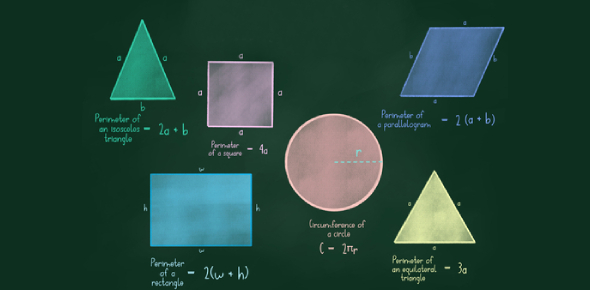# Area And Perimeter Quiz For Grade 6

10 Questions | Attempts: 14516
ShareSettingsAre you in 6th grade? Or, are you someone who just loves math? If yes, then here is an amazing area and perimeter quiz for grade 6 that we have brought for you. The quiz will test your knowledge of the area as well as the perimeter of an object. The quiz will also give a better understanding of these topics to you. We hope you give your best in this quiz and get a good score. Best of luck!

• 1.
What do we mean when we talk about Area?
• A.

The amount of surface/space that is covered

• B.

The type of object it is

• C.

The space around an object

• D.

How many sides an object has

• 2.
How do we find the Area of an object?
• A.

Using a ruler

• B.

Guessing

• C.

Length x width

• D.

1/2 base x height

• 3.
If a rectangle has : length= 12cm, Width = 4cm then its area is?
• A.

16cm2

• B.

48cm2

• C.

48cm

• D.

None of these

• 4.
What is the area of 6 square?
• A.

24

• B.

30

• C.

36

• D.

60

• 5.
What is the perimeter of a rectangle that has an L of 13 inches and a W of 5 inches?
• A.

36 inches

• B.

65 inches

• C.

85 inches

• D.

89 inches

• 6.
What is the area of a square 5 by 5 ft.?
• A.

12

• B.

13

• C.

25

• D.

25 sq. ft.

• 7.
What is the area of a square 9 by 9 ft.?
• A.

81

• B.

81 sq. ft.

• C.

122

• D.

100

• 8.
What is the perimeter of a rectangle 6 by 21 ft?
• A.

56

• B.

65

• C.

56 ft.

• D.

72 ft.

• E.

54 ft.

• 9.
What is the perimeter of a rectangle 6 by 21 ft?
• A.

56

• B.

65

• C.

56 ft.

• D.

72 ft.

• E.

54 ft.

• 10.
How do you find the perimeter of an object?
• A.

Add up one side two times.

• B.

Add up all of the sides.

• C.

Multiply all of the sides.

• D.

Multiply two of the sides.

• E.

None of the above

## Related TopicsBack to top
×

Wait!
Here's an interesting quiz for you.